Theory and Modern Applications

# Fuzzy ∗-homomorphisms and fuzzy ∗-derivations in induced fuzzy ${C}^{\ast }$-algebras

## Abstract

In this paper, we prove the Ulam-Hyers-Rassias stability of the Cauchy-Jensen additive functional equation

$f\left(\frac{x+y+z}{2}\right)+f\left(\frac{x-y+z}{2}\right)=f\left(x\right)+f\left(z\right)$

in fuzzy Banach spaces.

MSC:39B52, 46S40, 26E50, 46L05, 39B72.

## 1 Introduction

The stability problem of functional equations originated from the question of Ulam  concerning the stability of group homomorphisms. Hyers  gave the first affirmative partial answer to the question of Ulam for Banach spaces. Hyers’ theorem was generalized by Th.M. Rassias  for linear mappings by considering an unbounded Cauchy difference.

Theorem 1.1 (Rassias )

Let $f:E\to {E}^{\prime }$ be a mapping from a normed vector space E into a Banach space ${E}^{\prime }$ subject to the inequality $\parallel f\left(x+y\right)-f\left(x\right)-f\left(y\right)\parallel \le ϵ\left({\parallel x\parallel }^{p}+{\parallel y\parallel }^{p}\right)$ for all $x,y\in E$, where ϵ and p are constants with $ϵ>0$ and $0\le p<1$. Then the limit $L\left(x\right)={lim}_{n\to \mathrm{\infty }}\frac{f\left({2}^{n}x\right)}{{2}^{n}}$ exists for all $x\in E$ and $L:E\to {E}^{\prime }$ is the unique additive mapping which satisfies

$\parallel f\left(x\right)-L\left(x\right)\parallel \le \frac{2ϵ}{2-{2}^{p}}{\parallel x\parallel }^{p}$

for all $x\in E$. Also, if for each $x\in E$ the function $f\left(tx\right)$ is continuous in $t\in \mathbb{R}$, then L is linear.

The functional equation $f\left(x+y\right)+f\left(x-y\right)=2f\left(x\right)+2f\left(y\right)$ is called a quadratic functional equation. In particular, every solution of the quadratic functional equation is said to be a quadratic mapping. The Ulam-Hyers-Rassias stability of the quadratic functional equation was proved by Skof  for mappings $f:X\to Y$, where X is a normed space and Y is a Banach space. Cholewa  noticed that the theorem of Skof is still true if the relevant domain X is replaced by an Abelian group. Czerwik  proved the Ulam-Hyers-Rassias stability of the quadratic functional equation.

The stability problems of several functional equations have been extensively investigated by a number of authors, and there are many interesting results concerning this problem (see ).

Katsaras  defined a fuzzy norm on a vector space to construct a fuzzy vector topological structure on the space. Some mathematicians have defined fuzzy norms on a vector space from various points of view (see [13, 23, 24]).

In particular, Bag and Samanta , following Cheng and Mordeson , gave an idea of a fuzzy norm in such a manner that the corresponding fuzzy metric is of Karmosil and Michalek type . They established a decomposition theorem of a fuzzy norm into a family of crisp norms and investigated some properties of fuzzy normed spaces .

In this paper we consider a mapping $f:X\to Y$ satisfying the following Cauchy-Jensen functional equation

$f\left(\frac{x+y+z}{2}\right)+f\left(\frac{x-y+z}{2}\right)=f\left(x\right)+f\left(z\right)$
(1.1)

for all $x,y,z\in X$ and establish the fuzzy -homomorphisms and fuzzy -derivations of (1.1) in induced fuzzy ${C}^{\ast }$-algebras.

## 2 Preliminaries

Definition 2.1 Let X be a real vector space. A function $N:X×\mathbb{R}\to \left[0,1\right]$ is called a fuzzy norm on X if for all $x,y\in X$ and all $s,t\in \mathbb{R}$,

(N 1) $N\left(x,t\right)=0$ for $t\le 0$;

(N 2) $x=0$ if and only if $N\left(x,t\right)=1$ for all $t>0$;

(N 3) $N\left(cx,t\right)=N\left(x,\frac{t}{|c|}\right)$ if $c\ne 0$;

(N 4) $N\left(x+y,c+t\right)\ge min\left\{N\left(x,s\right),N\left(y,t\right)\right\}$;

(N 5) $N\left(x,\cdot \right)$ is a non-decreasing function of $\mathbb{R}$ and ${lim}_{t\to \mathrm{\infty }}N\left(x,t\right)=1$;

(N 6) for $x\ne 0$, $N\left(x,\cdot \right)$ is continuous on $\mathbb{R}$.

Example 2.1 Let $\left(X,\parallel \cdot \parallel \right)$ be a normed linear space and $\alpha ,\beta >0$. Then

$N\left(x,t\right)=\left\{\begin{array}{cc}\frac{\alpha t}{\alpha t+\beta \parallel x\parallel },\hfill & t>0,x\in X,\hfill \\ 0,\hfill & t\le 0,x\in X\hfill \end{array}$

is a fuzzy norm on X.

Definition 2.2 Let $\left(X,N\right)$ be a fuzzy normed vector space. A sequence $\left\{{x}_{n}\right\}$ in X is said to be convergent or converge if there exists an $x\in X$ such that ${lim}_{t\to \mathrm{\infty }}N\left({x}_{n}-x,t\right)=1$ for all $t>0$. In this case, x is called the limit of the sequence $\left\{{x}_{n}\right\}$ in X and we denote it by $N\text{-}{lim}_{t\to \mathrm{\infty }}{x}_{n}=x$.

Definition 2.3 Let $\left(X,N\right)$ be a fuzzy normed vector space. A sequence $\left\{{x}_{n}\right\}$ in X is called Cauchy if for each $ϵ>0$ and each $t>0$ there exists an ${n}_{0}\in \mathbb{N}$ such that for all $n\ge {n}_{0}$ and all $p>0$, we have $N\left({x}_{n+p}-{x}_{n},t\right)>1-ϵ$.

It is well known that every convergent sequence in a fuzzy normed vector space is Cauchy. If each Cauchy sequence is convergent, then the fuzzy norm is said to be complete and the fuzzy normed vector space is called a fuzzy Banach space.

We say that a mapping $f:X\to Y$ between fuzzy normed vector spaces X and Y is continuous at a point $x\in X$ if for each sequence $\left\{{x}_{n}\right\}$ converging to ${x}_{0}\in X$ the sequence $\left\{f\left({x}_{n}\right)\right\}$ converges to $f\left({x}_{0}\right)$. If $f:X\to Y$ is continuous at each $x\in X$, then $f:X\to Y$ is said to be continuous on X (see ).

Definition 2.4 Let X be a -algebra and $\left(X,N\right)$ a fuzzy normed space.

1. (1)

The fuzzy normed space $\left(X,N\right)$ is called a fuzzy normed -algebra if

$N\left(xy,st\right)\ge N\left(x,s\right)\cdot N\left(y,t\right),\phantom{\rule{2em}{0ex}}N\left({x}^{\ast },t\right)=N\left(x,t\right)$

for all $x,y\in X$ and all positive real numbers s and t.

2. (2)

A complete fuzzy normed -algebra is called a fuzzy Banach -algebra.

Example 2.2 Let $\left(X,\parallel \cdot \parallel \right)$ be a normed -algebra. Let

$N\left(x,t\right)=\left\{\begin{array}{cc}\frac{t}{t+\parallel x\parallel },\hfill & t>0,x\in X,\hfill \\ 0,\hfill & t\le 0,x\in X.\hfill \end{array}$

Then $N\left(x,t\right)$ is a fuzzy norm on X and $\left(X,N\right)$ is a fuzzy normed -algebra.

Definition 2.5 Let $\left(X,\parallel \cdot \parallel \right)$ be a normed ${C}^{\ast }$-algebra and ${N}_{x}$ a fuzzy norm on X.

1. (1)

The fuzzy normed -algebra $\left(X,{N}_{x}\right)$ is called an induced fuzzy normed -algebra.

2. (2)

The fuzzy Banach -algebra $\left(X,{N}_{x}\right)$ is called an induced fuzzy ${C}^{\ast }$-algebra.

Definition 2.6 Let $\left(X,{N}_{x}\right)$ and $\left(Y,N\right)$ be induced fuzzy normed -algebras.

1. (1)

A multiplicative $\mathbb{C}$-linear mapping $H:\left(X,{N}_{x}\right)\to \left(Y,N\right)$ is called a fuzzy -homomorphism if $H\left({x}^{\ast }\right)=H{\left(x\right)}^{\ast }$ for all $x\in X$.

2. (2)

A $\mathbb{C}$-linear mapping $D:\left(X,{N}_{x}\right)\to \left(X,{N}_{x}\right)$ is called a fuzzy -derivation if $D\left(xy\right)=D\left(x\right)y+xD\left(y\right)$ and $D\left({x}^{\ast }\right)=D{\left(x\right)}^{\ast }$ for all $x,y\in X$.

Definition 2.7 Let X be a set. A function $d:X×X\to \left[0,\mathrm{\infty }\right]$ is called a generalized metric on X if d satisfies the following conditions:

1. (1)

$d\left(x,y\right)=0$ if and only if $x=y$ for all $x,y\in X$;

2. (2)

$d\left(x,y\right)=d\left(y,x\right)$ for all $x,y\in X$;

3. (3)

$d\left(x,z\right)\le d\left(x,y\right)+d\left(y,z\right)$ for all $x,y,z\in X$.

Theorem 2.1 Let (X,d) be a complete generalized metric space and $J:X\to X$ be a strictly contractive mapping with Lipschitz constant $L<1$. Then, for all $x\in X$, either $d\left({J}^{n}x,{J}^{n+1}x\right)=\mathrm{\infty }$ for all nonnegative integers n or there exists a positive integer ${n}_{0}$ such that

1. (1)

$d\left({J}^{n}x,{J}^{n+1}x\right)<\mathrm{\infty }$ for all ${n}_{0}\ge {n}_{0}$;

2. (2)

the sequence $\left\{{J}^{n}x\right\}$ converges to a fixed point ${y}^{\ast }$ of J;

3. (3)

${y}^{\ast }$ is the unique fixed point of J in the set $Y=\left\{y\in X:d\left({J}^{{n}_{0}}x,y\right)<\mathrm{\infty }\right\}$;

4. (4)

$d\left(y,{y}^{\ast }\right)\le \frac{1}{1-L}d\left(y,Jy\right)$ for all $y\in Y$.

## 3 Hyers-Ulam-Rassias stability of CJA functional equation (1.1) in fuzzy Banach ∗-algebras

In this section, using the fixed point alternative approach we prove the Ulam-Hyers-Rassias stability of the functional equation (1.1) in fuzzy Banach spaces. Throughout this paper, assume that X is a vector space and that $\left(Y,N\right)$ is a fuzzy Banach space.

Theorem 3.1 Let $\phi :{X}^{3}\to \left[0,\mathrm{\infty }\right)$ be a function such that there exists an $L<\frac{1}{2}$ with $\phi \left(\frac{x}{2},\frac{y}{2},\frac{z}{2}\right)\le \frac{L\phi \left(x,y,z\right)}{2}$ for all $x,y,z\in X$. Let $f:X\to Y$ be a mapping satisfying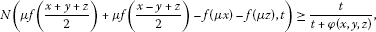(3.1)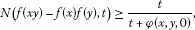(3.2)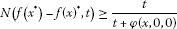(3.3)

for all $x,y,z\in X$ and $t>0$. Then there exists a fuzzy -homomorphism $H:X\to Y$ such that

$N\left(f\left(x\right)-H\left(x\right),t\right)\ge \frac{\left(2-2L\right)t}{\left(2-2L\right)t+L\phi \left(x,2x,x\right)}$
(3.4)

for all $x\in X$ and $t>0$.

Proof Letting $\mu =1$ and replacing $\left(x,y,z\right)$ by $\left(x,2x,x\right)$ in (3.1), we have

$N\left(f\left(2x\right)-2f\left(x\right),t\right)\ge \frac{t}{t+\phi \left(x,2x,x\right)}$
(3.5)

for all $x\in X$ and $t>0$. Replacing x by $\frac{x}{2}$ in (3.5), we obtain

$N\left(f\left(x\right)-2f\left(\frac{x}{2}\right),t\right)\ge \frac{t}{t+\phi \left(\frac{x}{2},x,\frac{x}{2}\right)}\ge \frac{t}{t+\frac{L}{2}\phi \left(x,2x,x\right)}.$
(3.6)

Consider the set $S:=\left\{g:X\to Y\right\}$ and the generalized metric d in S defined by

$d\left(f,g\right)=inf\left\{\mu \in {\mathbb{R}}^{+}:N\left(g\left(x\right)-h\left(x\right),\mu t\right)\ge \frac{t}{t+\phi \left(x,2x,x\right)},\mathrm{\forall }x\in X,t>0\right\},$

where $inf\mathrm{\varnothing }=+\mathrm{\infty }$. It is easy to show that $\left(S,d\right)$ is complete (see ). Now, we consider a linear mapping $J:S\to S$ such that $Jg\left(x\right):=2g\left(\frac{x}{2}\right)$ for all $x\in X$. Let $g,h\in S$ be such that $d\left(g,h\right)=ϵ$. Then $N\left(g\left(x\right)-h\left(x\right),ϵt\right)\ge \frac{t}{t+\phi \left(x,2x,x\right)}$ for all $x\in X$ and $t>0$. Hence

$\begin{array}{rcl}N\left(Jg\left(x\right)-Jh\left(x\right),Lϵt\right)& =& N\left(2g\left(\frac{x}{2}\right)-2h\left(\frac{x}{2}\right),Lϵt\right)=N\left(g\left(\frac{x}{2}\right)-h\left(\frac{x}{2}\right),\frac{Lϵt}{2}\right)\\ \ge & \frac{\frac{Lt}{2}}{\frac{Lt}{2}+\phi \left(\frac{x}{2},x,\frac{x}{2}\right)}\ge \frac{\frac{Lt}{2}}{\frac{Lt}{2}+\frac{L\phi \left(x,2x,x\right)}{2}}=\frac{t}{t+\phi \left(x,2x,x\right)}\end{array}$

for all $x\in X$ and $t>0$. Thus $d\left(g,h\right)=ϵ$ implies that $d\left(Jg,Jh\right)\le Lϵ$. This means that $d\left(Jg,Jh\right)\le Ld\left(g,h\right)$ for all $g,h\in S$. It follows from (3.6) that

$N\left(2f\left(\frac{x}{2}\right)-f\left(x\right),\frac{Lt}{2}\right)\ge \frac{t}{t+\phi \left(x,2x,x\right)}$

for all $x\in X$ and all $t>0$. This implies that $d\left(f,Jf\right)\le \frac{L}{2}$. By Theorem 2.1, there exists a mapping $H:X\to Y$ satisfying the following:

1. (1)

H is a fixed point of J, that is,

$H\left(\frac{x}{2}\right)=\frac{H\left(x\right)}{2}$
(3.7)

for all $x\in X$. The mapping H is a unique fixed point of J in the set $\mathrm{\Omega }=\left\{h\in S:d\left(g,h\right)<\mathrm{\infty }\right\}$. This implies that H is a unique mapping satisfying (3.7) such that there exists $\mu \in \left(0,\mathrm{\infty }\right)$ satisfying $N\left(f\left(x\right)-H\left(x\right),\mu t\right)\ge \frac{t}{t+\phi \left(x,2x,x\right)}$ for all $x\in X$ and $t>0$.

2. (2)

$d\left({J}^{n}f,H\right)\to 0$ as $n\to \mathrm{\infty }$. This implies the equality

$N\text{-}\underset{n\to \mathrm{\infty }}{lim}{2}^{n}f\left(\frac{x}{{2}^{n}}\right)=H\left(x\right)$
(3.8)

for all $x\in X$.

3. (3)

$d\left(f,H\right)\le \frac{d\left(f,Jf\right)}{1-L}$ with $f\in \mathrm{\Omega }$, which implies the inequality $d\left(f,H\right)\le \frac{L}{2-2L}$. This implies that the inequality (3.4) holds. Furthermore, it follows from (3.1) and (3.8) that

$\begin{array}{c}N\left(\mu H\left(\frac{x+y+z}{2}\right)+\mu H\left(\frac{x-y+z}{2}\right)-H\left(\mu x\right)-H\left(\mu z\right),t\right)\hfill \\ \phantom{\rule{1em}{0ex}}=N\text{-}\underset{n\to \mathrm{\infty }}{lim}\left({2}^{n}\mu f\left(\frac{x+y+z}{{2}^{n+1}}\right)+{2}^{n}\mu f\left(\frac{x-y+z}{{2}^{n+1}}\right)-{2}^{n}f\left(\frac{\mu x}{{2}^{n}}\right)-{2}^{n}f\left(\frac{\mu z}{{2}^{n}}\right),t\right)\hfill \\ \phantom{\rule{1em}{0ex}}\ge \underset{n\to \mathrm{\infty }}{lim}\frac{\frac{t}{{2}^{n}}}{\frac{t}{{2}^{n}}+\phi \left(\frac{x}{{2}^{n}},\frac{y}{{2}^{n}},\frac{z}{{2}^{n}}\right)}\ge \underset{n\to \mathrm{\infty }}{lim}\frac{\frac{t}{{2}^{n}}}{\frac{t}{{2}^{n}}+\frac{{L}^{n}}{{2}^{n}}\phi \left(x,y,z\right)}\to 1\hfill \end{array}$

for all $x,y,z\in X$, all $t>0$ and all $\mu \in \mathbb{C}$. Hence

$\mu H\left(\frac{x+y+z}{2}\right)+\mu H\left(\frac{x-y+z}{2}\right)-H\left(\mu x\right)-H\left(\mu z\right)=0$

for all $x,y,z\in X$. So the mapping $H:X\to Y$ is additive and $\mathbb{C}$-linear. By (3.2),

$N\left({4}^{n}f\left(\frac{xy}{{4}^{n}}\right)-{2}^{n}f\left(\frac{x}{{2}^{n}}\right)\cdot {2}^{n}f\left(\frac{y}{{2}^{n}}\right),{4}^{n}t\right)\ge \frac{t}{t+\phi \left(\frac{x}{{2}^{n}},\frac{y}{{2}^{n}},0\right)}$

for all $x,y\in X$ and all $t>0$. Then

for all $x,y\in X$ and all $t>0$. So $N\left(H\left(xy\right)-H\left(x\right)H\left(y\right),t\right)=1$ for all $x,y\in X$ and all $t>0$. By (3.3)

$N\left({2}^{n}f\left(\frac{{x}^{\ast }}{{2}^{n}}\right)-{2}^{n}f{\left(\frac{x}{{2}^{n}}\right)}^{\ast },{2}^{n}t\right)\ge \frac{t}{t+\phi \left(\frac{x}{{2}^{n}},0,0\right)}$

for all $x\in X$ and all $t>0$. So

$N\left({2}^{n}f\left(\frac{{x}^{\ast }}{{2}^{n}}\right)-{2}^{n}f{\left(\frac{x}{{2}^{n}}\right)}^{\ast },t\right)\ge \frac{\frac{t}{{2}^{n}}}{\frac{t}{{2}^{n}}+\phi \left(\frac{x}{{2}^{n}},0,0\right)}\ge \frac{\frac{t}{{2}^{n}}}{\frac{t}{{2}^{n}}+\frac{{L}^{n}}{{2}^{n}}\phi \left(x,0,0\right)}$

for all $x\in X$ and all $t>0$. Since ${lim}_{n\to +\mathrm{\infty }}\frac{\frac{t}{{2}^{n}}}{\frac{t}{{2}^{n}}+\frac{{L}^{n}}{{2}^{n}}\phi \left(x,0,0\right)}=1$, for all $x\in X$ and $t>0$, we get $N\left(H\left({x}^{\ast }\right)-H{\left(x\right)}^{\ast },t\right)=1$ for all $x\in X$ and all $t>0$. Thus $H\left({x}^{\ast }\right)=H{\left(x\right)}^{\ast }$ for all $x\in X$. □

Theorem 3.2 Let $\phi :{X}^{3}\to \left[0,\mathrm{\infty }\right)$ be a function such that there exists an $L<1$ with $\phi \left(x,y,z\right)\le 2L\phi \left(\frac{x}{2},\frac{y}{2},\frac{z}{2}\right)$ for all $x,y,z\in X$. Let $f:X\to Y$ be a mapping satisfying (3.1)-(3.3). Then the limit $H\left(x\right):=N\text{-}{lim}_{n\to \mathrm{\infty }}\frac{f\left({2}^{n}x\right)}{{2}^{n}}$ exists for each $x\in X$ and defines a fuzzy -homomorphism $H:X\to Y$ such that

$N\left(f\left(x\right)-H\left(x\right),t\right)\ge \frac{\left(2-2L\right)t}{\left(2-2L\right)t+\phi \left(x,2x,x\right)}$
(3.9)

for all $x\in X$ and all $t>0$.

Proof Let $\left(S,d\right)$ be a generalized metric space defined as in the proof of Theorem 3.1. Consider the linear mapping $J:S\to S$ such that $Jg\left(x\right):=\frac{g\left(2x\right)}{2}$ for all $x\in X$. Let $g,h\in S$ be such that $d\left(g,h\right)=ϵ$. Then $N\left(g\left(x\right)-h\left(x\right),ϵt\right)\ge \frac{t}{t+\phi \left(x,2x,x\right)}$ for all $x\in X$ and $t>0$. Hence

$\begin{array}{rcl}N\left(Jg\left(x\right)-Jh\left(x\right),Lϵt\right)& =& N\left(\frac{g\left(2x\right)}{2}-\frac{h\left(2x\right)}{2},Lϵt\right)=N\left(g\left(2x\right)-h\left(2x\right),2Lϵt\right)\\ \ge & \frac{2Lt}{2Lt+\phi \left(2x,,4x,2x\right)}\ge \frac{2Lt}{2Lt+2L\phi \left(x,,2x,x\right)}\\ =& \frac{t}{t+\phi \left(x,2x,x\right)}\end{array}$

for all $x\in X$ and $t>0$. Thus $d\left(g,h\right)=ϵ$ implies that $d\left(Jg,Jh\right)\le Lϵ$. This means that $d\left(Jg,Jh\right)\le Ld\left(g,h\right)$ for all $g,h\in S$. It follows from (3.5) that

$N\left(\frac{f\left(2x\right)}{2}-f\left(x\right),\frac{t}{2}\right)\ge \frac{t}{t+\phi \left(x,2x,x\right)}$
(3.10)

for all $x\in X$ and $t>0$. So $d\left(f,Jf\right)\le \frac{1}{2}$. By Theorem 2.1, there exists a mapping $H:X\to Y$ satisfying the following:

1. (1)

H is a fixed point of J, that is,

$2H\left(x\right)=H\left(2x\right)$
(3.11)

for all $x\in X$. The mapping H is a unique fixed point of J in the set $\mathrm{\Omega }=\left\{h\in S:d\left(g,h\right)<\mathrm{\infty }\right\}$. This implies that H is a unique mapping satisfying (3.11) such that there exists $\mu \in \left(0,\mathrm{\infty }\right)$ satisfying $N\left(f\left(x\right)-H\left(x\right),\mu t\right)\ge \frac{t}{t+\phi \left(x,2x,x\right)}$ for all $x\in X$ and $t>0$.

2. (2)

$d\left({J}^{n}f,H\right)\to 0$ as $n\to \mathrm{\infty }$. This implies the equality $H\left(x\right)=N\text{-}{lim}_{n\to \mathrm{\infty }}\frac{f\left({2}^{n}x\right)}{{2}^{n}}$ for all $x\in X$.

3. (3)

$d\left(f,H\right)\le \frac{d\left(f,Jf\right)}{1-L}$ with $f\in \mathrm{\Omega }$, which implies the inequality $d\left(f,H\right)\le \frac{1}{2-2L}$. This implies that the inequality (3.9) holds. The rest of the proof is similar to that of the proof of Theorem 3.1. □

## 4 Hyers-Ulam-Rassias stability of CJA functional equation (1.1) in induced fuzzy ${C}^{\ast }$-algebras

Throughout this section, assume that X is a unital ${C}^{\ast }$-algebra with unit e and unitary group $\mathcal{U}\left(X\right):=\left\{u\in X:{u}^{\ast }u=u{u}^{\ast }=e\right\}$ and that Y is a unital ${C}^{\ast }$-algebra.

Using the fixed point method, we prove the Hyers-Ulam-Rassias stability of the Cauchy-Jensen additive functional equation (1.1) in induced fuzzy ${C}^{\ast }$-algebras.

Theorem 4.1 Let $\phi :{X}^{3}\to \left[0,\mathrm{\infty }\right)$ be a function such that there exists an $L<\frac{1}{2}$ with $\phi \left(\frac{x}{2},\frac{y}{2},\frac{z}{2}\right)\le \frac{L\phi \left(x,y,z\right)}{2}$ for all $x,y,z\in X$. Let $f:X\to Y$ be a mapping satisfying (3.1) and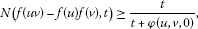(4.1)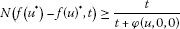(4.2)

for all $u,v\in \mathcal{U}\left(X\right)$ and all $t>0$. Then there exists a fuzzy -homomorphism $H:X\to Y$ satisfying (3.4).

Proof By the same reasoning as in the proof of Theorem 3.1, there is a $\mathbb{C}$-linear mapping $H:X\to Y$ satisfying (3.4). The mapping $H:X\to Y$ is given by

$N\text{-}\underset{p\to \mathrm{\infty }}{lim}{2}^{n}f\left(\frac{x}{{2}^{n}}\right)=H\left(x\right)$

for all $x\in X$. By (4.1),

$N\left({4}^{n}f\left(\frac{uv}{{4}^{n}}\right)-{2}^{n}f\left(\frac{u}{{2}^{n}}\right)\cdot {2}^{n}f\left(\frac{v}{{2}^{n}}\right),{4}^{n}t\right)\ge \frac{t}{t+\phi \left(\frac{u}{{2}^{n}},\frac{v}{{2}^{n}},0\right)}$

for all $u,v\in \mathcal{U}\left(X\right)$ and all $t>0$. Then

for all $x,y\in \mathcal{U}\left(X\right)$ and all $t>0$. So $N\left(H\left(uv\right)-H\left(u\right)H\left(v\right),t\right)=1$ for all $u,v\in \mathcal{U}\left(X\right)$ and all $t>0$. Therefore

$H\left(uv\right)=H\left(u\right)H\left(v\right),$
(4.3)

for all $u,v\in \mathcal{U}\left(X\right)$. Since H is $\mathbb{C}$-linear and each $x\in X$ is a finite linear combination of unitary elements, i.e.,

$x=\sum _{j=1}^{m}{\lambda }_{j}{u}_{j}\left({\lambda }_{j}\in \mathbb{C},{u}_{j}\in U\left(X\right)\right),$

it follows from (4.3) that

$H\left(xv\right)=H\left(\sum _{j=1}^{m}{\lambda }_{j}{u}_{j}v\right)=\sum _{j=1}^{n}{\lambda }_{j}H\left({u}_{j}v\right)=\sum _{j=1}^{n}{\lambda }_{j}H\left({u}_{j}\right)H\left(v\right)=H\left(\sum _{j=1}^{m}{\lambda }_{j}{u}_{j}\right)H\left(v\right)$

for all $v\in \mathcal{U}\left(X\right)$. So $H\left(xv\right)=H\left(x\right)H\left(v\right)$. Similarly, one can obtain that $H\left(xy\right)=H\left(x\right)H\left(y\right)$ for all $x,y\in X$. By (4.2)

$N\left({2}^{n}f\left(\frac{{u}^{\ast }}{{2}^{n}}\right)-{2}^{n}f{\left(\frac{u}{{2}^{n}}\right)}^{\ast },{2}^{n}t\right)\ge \frac{t}{t+\phi \left(\frac{u}{{2}^{n}},0,0\right)}$

for all $u\in \mathcal{U}\left(X\right)$ and all $t>0$. So

$N\left({2}^{n}f\left(\frac{{u}^{\ast }}{{2}^{n}}\right)-{2}^{n}f{\left(\frac{u}{{2}^{n}}\right)}^{\ast },t\right)\ge \frac{\frac{t}{{2}^{n}}}{\frac{t}{{2}^{n}}+\phi \left(\frac{u}{{2}^{n}},0,0\right)}\ge \frac{\frac{t}{{2}^{n}}}{\frac{t}{{2}^{n}}+\frac{{L}^{n}}{{2}^{n}}\phi \left(u,0,0\right)}$

for all $u\in \mathcal{U}\left(X\right)$ and all $t>0$. Since ${lim}_{n\to +\mathrm{\infty }}\frac{\frac{t}{{2}^{n}}}{\frac{t}{{2}^{n}}+\frac{{L}^{n}}{{2}^{n}}\phi \left(u,0,0\right)}=1$, for all $u\in \mathcal{U}\left(X\right)$ and $t>0$ , we get $N\left(H\left({u}^{\ast }\right)-H{\left(u\right)}^{\ast },t\right)=1$ for all $u\in \mathcal{U}\left(X\right)$ and all $t>0$. Thus

$H\left({u}^{\ast }\right)=H{\left(u\right)}^{\ast }$
(4.4)

for all $u\in \mathcal{U}\left(X\right)$. Since H is $\mathbb{C}$-linear, i.e., $x\in X$ is a finite linear combination of unitary elements, i.e., $x={\sum }_{j=1}^{m}{\lambda }_{j}{u}_{j}$ (${\lambda }_{j}\in \mathbb{C}$, ${u}_{j}\in \mathcal{U}\left(X\right)$), it follows from (4.4) that

$H\left({x}^{\ast }\right)=H\left(\sum _{j=1}^{m}\overline{{\lambda }_{j}}{u}_{j}^{\ast }\right)=\sum _{j=1}^{n}\overline{{\lambda }_{j}}H\left({u}_{j}^{\ast }\right)=\sum _{j=1}^{n}\overline{{\lambda }_{j}}H{\left({u}_{j}\right)}^{\ast }=H{\left(\sum _{j=1}^{m}{\lambda }_{j}{u}_{j}\right)}^{\ast }=H{\left(x\right)}^{\ast }$

for all $x\in X$. So $H\left({x}^{\ast }\right)=H{\left(x\right)}^{\ast }$ for all $x\in X$. Therefore, the mapping $H:X\to Y$ is a -homomorphism. □

Similarly, we have the following. We will omit the proof.

Theorem 4.2 Let $\phi :{X}^{3}\to \left[0,\mathrm{\infty }\right)$ be a function such that there exists an $L<1$ with $\phi \left(x,y,z\right)\le 2L\phi \left(\frac{x}{2},\frac{y}{2},\frac{z}{2}\right)$ for all $x,y,z\in X$. Let $f:X\to Y$ be a mapping satisfying (3.1), (4.1) and (4.2). Then the limit $H\left(x\right):=N\text{-}{lim}_{n\to \mathrm{\infty }}\frac{f\left({2}^{n}x\right)}{{2}^{n}}$ exists for each $x\in X$ and defines a fuzzy -homomorphism $H:X\to Y$ such that

$N\left(f\left(x\right)-H\left(x\right),t\right)\ge \frac{\left(2-2L\right)t}{\left(2-2L\right)t+\phi \left(x,2x,x\right)}$
(4.5)

for all $x\in X$ and all $t>0$.

## 5 Hyers-Ulam-Rassias stability of fuzzy ∗-derivations in fuzzy Banach ∗-algebras and in induced fuzzy ${C}^{\ast }$-algebras

In this section, assume that $\left(X,{N}_{X}\right)$ is a fuzzy Banach -algebra. Using the fixed point method, we prove the Hyers-Ulam-Rassias stability of fuzzy -derivations in fuzzy Banach -algebras.

Theorem 5.1 Let $\phi :{X}^{2}\to \left[0,\mathrm{\infty }\right)$ be a function such that there exists an $L<\frac{1}{2}$ with $\phi \left(\frac{x}{2},\frac{y}{2},\frac{z}{2}\right)\le \frac{L\phi \left(x,y,z\right)}{2}$ for all $x,y,z\in X$. Let $f:X\to X$ be a mapping satisfying (3.1), (3.3) and

${N}_{X}\left(f\left(xy\right)-xf\left(y\right)-yf\left(x\right),t\right)\ge \frac{t}{t+\phi \left(x,y,0\right)}$
(5.1)

for all $x,y\in X$ and all $t>0$. Then $\delta \left(x\right):=N\text{-}{lim}_{n\to \mathrm{\infty }}{2}^{n}f\left(\frac{x}{{2}^{n}}\right)$ exists for each $x\in X$ and defines a fuzzy -derivation $\delta :X\to X$ such that

$N\left(f\left(x\right)-\delta \left(x\right),t\right)\ge \frac{\left(2-2L\right)t}{\left(2-2L\right)t+L\phi \left(x,2x,x\right)}$
(5.2)

for all $x\in X$ and all $t>0$.

Proof The proof is similar to the proof of Theorem 3.1. □

Theorem 5.2 Let $\phi :{X}^{2}\to \left[0,\mathrm{\infty }\right)$ be a function such that there exists an $L<1$ with $\phi \left(x,y,z\right)\le 2L\phi \left(\frac{x}{2},\frac{y}{2},\frac{z}{2}\right)$ for all $x,y,z\in X$. Let $f:X\to Y$ be a mapping satisfying (3.1) and (5.1). Then the limit $\delta \left(x\right):=N\text{-}{lim}_{p\to \mathrm{\infty }}\frac{f\left({2}^{n}x\right)}{{2}^{n}}$ exists for each $x\in X$ and defines a fuzzy -derivation $\delta :X\to Y$ such that

$N\left(f\left(x\right)-\delta \left(x\right),t\right)\ge \frac{\left(2-2L\right)t}{\left(2-2L\right)t+\phi \left(x,2x,x\right)}$
(5.3)

for all $x\in X$ and all $t>0$.

## References

1. Ulam SM: Problems in Modern Mathematics. Wiley, New York; 1964.

2. Hyers DH: On the stability of the linear functional equation. Proc. Natl. Acad. Sci. USA 1941, 27: 222–224. 10.1073/pnas.27.4.222

3. Rassias TM: On the stability of the linear mapping in Banach spaces. Proc. Am. Math. Soc. 1978, 72: 297–300. 10.1090/S0002-9939-1978-0507327-1

4. Skof F: Local properties and approximation of operators. Rend. Semin. Mat. Fis. Milano 1983, 53: 113–129. 10.1007/BF02924890

5. Cholewa PW: Remarks on the stability of functional equations. Aequ. Math. 1984, 27: 76–86. 10.1007/BF02192660

6. Czerwik S: On the stability of the quadratic mapping in normed spaces. Abh. Math. Semin. Univ. Hamb. 1992, 62: 239–248.

7. Eshaghi Gordji M, Savadkouhi MB: Stability of mixed type cubic and quartic functional equations in random normed spaces. J. Inequal. Appl. 2009., 2009: Article ID 527462

8. Eshaghi Gordji M, Savadkouhi MB, Park C: Quadratic-quartic functional equations in RN-spaces. J. Inequal. Appl. 2009., 2009: Article ID 868423

9. Eshaghi Gordji M, Khodaei H: Stability of Functional Equations. Lap Lambert Academic Publishing, Saarbrücken; 2010.

10. Eshaghi Gordji M, Zolfaghari S, Rassias JM, Savadkouhi MB: Solution and stability of a mixed type cubic and quartic functional equation in quasi-Banach spaces. Abstr. Appl. Anal. 2009., 2009: Article ID 417473

11. Gǎvruta P: A generalization of the Hyers-Ulam-Rassias stability of approximately additive mappings. J. Math. Anal. Appl. 1994, 184: 431–436. 10.1006/jmaa.1994.1211

12. Park C: On the stability of the linear mapping in Banach modules. J. Math. Anal. Appl. 2002, 275: 711–720. 10.1016/S0022-247X(02)00386-4

13. Park C: Fuzzy stability of a functional equation associated with inner product spaces. Fuzzy Sets Syst. 2009, 160: 1632–1642. 10.1016/j.fss.2008.11.027

14. Park C: Generalized Hyers-Ulam-Rassias stability of n -sesquilinear-quadratic mappings on Banach modules over ${C}^{\ast }$ -algebras. J. Comput. Appl. Math. 2005, 180: 279–291. 10.1016/j.cam.2004.11.001

15. Park C: Fixed points and Hyers-Ulam-Rassias stability of Cauchy-Jensen functional equations in Banach algebras. Fixed Point Theory Appl. 2007., 2007: Article ID 50175

16. Park C: Generalized Hyers-Ulam-Rassias stability of quadratic functional equations: a fixed point approach. Fixed Point Theory Appl. 2008., 2008: Article ID 493751

17. Rassias TM: On the stability of the quadratic functional equation and its application. Stud. Univ. Babeş-Bolyai, Math. 1998, XLIII: 89–124.

18. Rassias TM: On the stability of functional equations in Banach spaces. J. Math. Anal. Appl. 2000, 251: 264–284. 10.1006/jmaa.2000.7046

19. Rassias TM, Šemrl P: On the Hyers-Ulam stability of linear mappings. J. Math. Anal. Appl. 1993, 173: 325–338. 10.1006/jmaa.1993.1070

20. Saadati R, Vaezpour M, Cho YJ: A note to paper ’On the stability of cubic mappings and quartic mappings in random normed spaces’. J. Inequal. Appl. 2009., 2009: Article ID 214530. doi:10.1155/2009/214530

21. Saadati R, Zohdi MM, Vaezpour SM: Nonlinear L -random stability of an ACQ functional equation. J. Inequal. Appl. 2011., 2011: Article ID 194394. doi:10.1155/2011/194394

22. Katsaras AK: Fuzzy topological vector spaces. Fuzzy Sets Syst. 1984, 12: 143–154. 10.1016/0165-0114(84)90034-4

23. Felbin C: Finite-dimensional fuzzy normed linear space. Fuzzy Sets Syst. 1992, 48: 239–248. 10.1016/0165-0114(92)90338-5

24. Krishna SV, Sarma KKM: Separation of fuzzy normed linear spaces. Fuzzy Sets Syst. 1994, 63: 207–217. 10.1016/0165-0114(94)90351-4

25. Bag T, Samanta SK: Finite dimensional fuzzy normed linear spaces. J. Fuzzy Math. 2003, 11: 687–705.

26. Cheng SC, Mordeson JN: Fuzzy linear operators and fuzzy normed linear spaces. Bull. Calcutta Math. Soc. 1994, 86: 429–436.

27. Karmosil I, Michalek J: Fuzzy metric and statistical metric spaces. Kybernetika 1975, 11: 326–334.

28. Bag T, Samanta SK: Fuzzy bounded linear operators. Fuzzy Sets Syst. 2005, 151: 513–547. 10.1016/j.fss.2004.05.004

29. Mihet D, Radu V: On the stability of the additive Cauchy functional equation in random normed spaces. J. Math. Anal. Appl. 2008, 343: 567–572. 10.1016/j.jmaa.2008.01.100

## Author information

Authors

### Corresponding author

Correspondence to H Azadi Kenary.

### Competing interests

The authors declare that they have no competing interests.

### Authors’ contributions

All authors conceived of the study, participated in its design and coordination, drafted the manuscript, participated in the sequence alignment, and read and approved the final manuscript.

## Rights and permissions

Open Access This article is distributed under the terms of the Creative Commons Attribution 2.0 International License (https://creativecommons.org/licenses/by/2.0), which permits unrestricted use, distribution, and reproduction in any medium, provided the original work is properly cited.

Reprints and Permissions

Azadi Kenary, H., Zohdi, A., Eshaghi Gordji, M. et al. Fuzzy -homomorphisms and fuzzy -derivations in induced fuzzy ${C}^{\ast }$-algebras. Adv Differ Equ 2012, 147 (2012). https://doi.org/10.1186/1687-1847-2012-147

• Accepted:

• Published:

• DOI: https://doi.org/10.1186/1687-1847-2012-147

### Keywords

• Hyers-Ulam-Rassias stability
• fixed point method
• fuzzy Banach -algebra
• induced fuzzy ${C}^{\ast }$-algebra